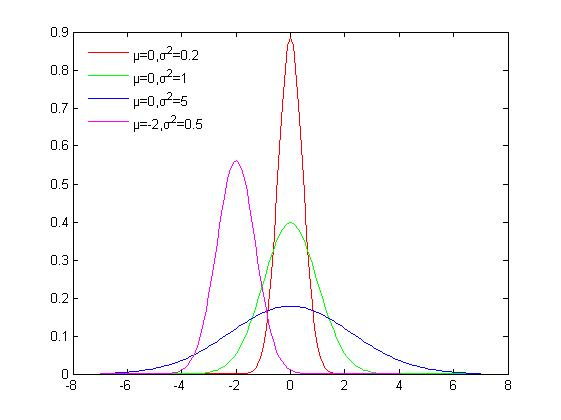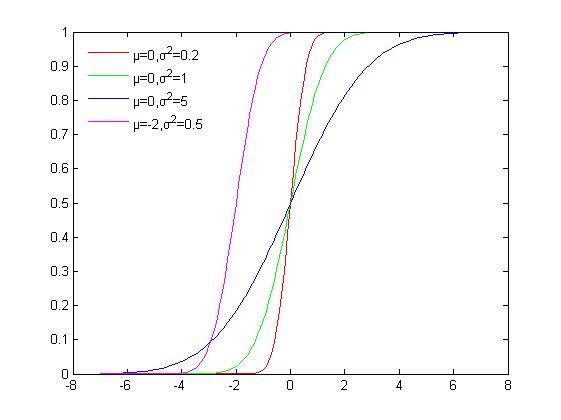# 高斯分布概率密度函数（PDF）和累积分布函数(CDF)$高斯分布概率密度函数（PDF）和累积分布函数(CDF)$$f(x;\mu,\sigma)=\frac{1}{\sigma\sqrt{2\pi}} \, \exp \left( -\frac{(x- \mu)^2}{2\sigma^2} \right)$

### 累积分布函数$高斯分布概率密度函数（PDF）和累积分布函数(CDF)$matlab实现代码
function [ output_args ] = Normpropogation( input_args )
%NORM PROPOGATION Summary of this function goes here
%   Detailed explanation goes here
x=-7:0.1:7;
y1=normpdf(x,0,1);
y2=normpdf(x,0,0.45);
y3=normpdf(x,0,2.23);
y4=normpdf(x,-2,0.71);
%线型，颜色，点型，线宽
figure;
plot(x,y2,'-r','LineWidth',1);
hold on;
plot(x,y1,'-g','LineWidth',1);
hold on;
plot(x,y3,'-b','LineWidth',1);
hold on;
plot(x,y4,'-m','LineWidth',1);
hold on;
% legend('','','','',1);
legend('μ=0,σ^2=0.2','μ=0,σ^2=1','μ=0,σ^2=5','μ=-2,σ^2=0.5',2);
legend boxoff;

figure;
z1=normcdf(x,0,0.45);
z2=normcdf(x,0,1);
z3=normcdf(x,0,2.23);
z4=normcdf(x,-2,0.71);
plot(x,z1,'-r',x,z2,'-g',x,z3,'-b',x,z4,'-m');
h=legend('μ=0,σ^2=0.2','μ=0,σ^2=1','μ=0,σ^2=5','μ=-2,σ^2=0.5',2);
legend boxoff;
end

http://www.tainingxinwen.cn/news/485401.html

### 精彩评论换一张

### 图文推荐